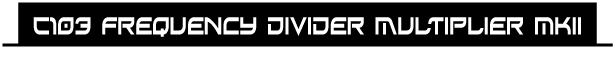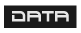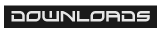The C103 Frequency Divider / Multiplier MKII includes two frequency dividers and two frequency multipliers. It generates four new signals which frequencies are one / two octaves higher / lower than the original input. This new MKII version, adds a lot of functionality to the original design. It has four built in mixers , new individual outputs and new voltage controlled distortion circuit with independent output. Additionally each divider and multiplier stage has his own level potentiometer. Thanks to these new additions the divider and multiplier circuits can be used independently. For example a VCO can be connected to the divider to use it as suboctave generator and a LFO can be connected to the multiplier to get synchronized modulations. The C103 Frequency Divider / Multiplier MKII can be used in many different ways : As suboctave Generator To create complex and rich sounds from a simple waveform. To create synchronized modulations As a clock divider As frequency multiplier To create complex modulation signals As a voltage controlled distortion unit As pattern generator If you like the original C103 , this new version retains the same functionality using the Switched mixer but it goes one step further. Thanks to the new level potentiometers , the divider and the multiplier of each stage can have different signal levels.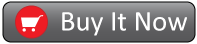Price : 255 € + VAT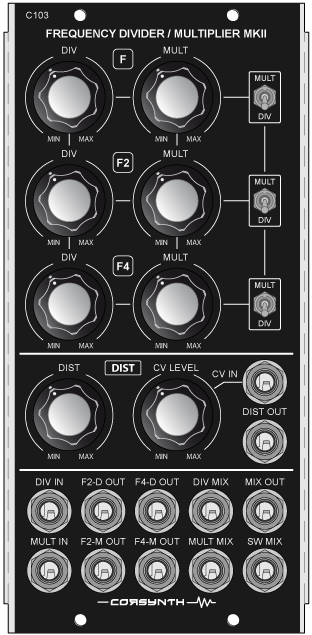The frequency divider input is normalized to the multiplier input, If nothing is connected to the divider input, it will take the signal connected to the multiplier input as its input signal.

The C103 Frequency Divider / Multiplier MKII is built using high quality electronic components, Switchcraft jacks, Cosmo knobs (the same used by Moog Modular) , anodized aluminum front panel….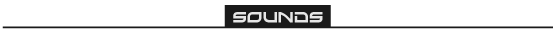Back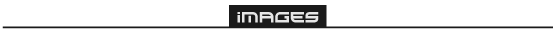Back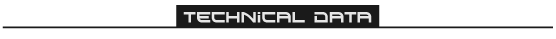• Module Format : 5U, MU format ( Synthesizers.com, Moog )
• Module Width : 2 MU ( Moog Unit )
• Module Depth : 52 mm ( 2,05 inches )
• Power : +15V@75mA , -15V@80mA
• Power connectors : Synthesizers.com , MOTM ( 4 pin )

Back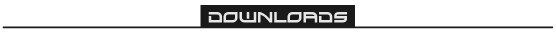Back